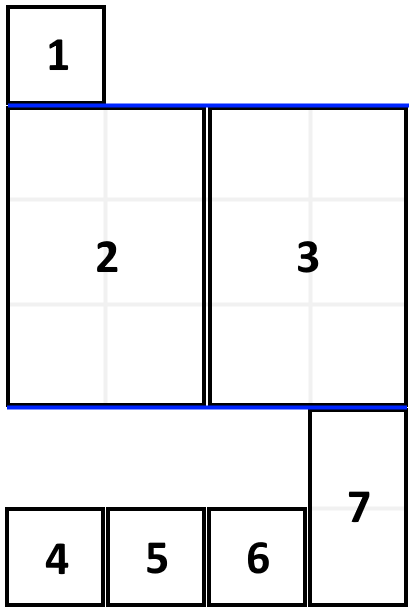## Algorithm

Problem Name: 1105. Filling Bookcase Shelves

You are given an array `books` where `books[i] = [thicknessi, heighti]` indicates the thickness and height of the `ith` book. You are also given an integer `shelfWidth`.

We want to place these books in order onto bookcase shelves that have a total width `shelfWidth`.

We choose some of the books to place on this shelf such that the sum of their thickness is less than or equal to `shelfWidth`, then build another level of the shelf of the bookcase so that the total height of the bookcase has increased by the maximum height of the books we just put down. We repeat this process until there are no more books to place.

Note that at each step of the above process, the order of the books we place is the same order as the given sequence of books.

• For example, if we have an ordered list of `5` books, we might place the first and second book onto the first shelf, the third book on the second shelf, and the fourth and fifth book on the last shelf.

Return the minimum possible height that the total bookshelf can be after placing shelves in this manner.

Example 1:```Input: books = [[1,1],[2,3],[2,3],[1,1],[1,1],[1,1],[1,2]], shelf_width = 4
Output: 6
Explanation:
The sum of the heights of the 3 shelves is 1 + 3 + 2 = 6.
Notice that book number 2 does not have to be on the first shelf.
```

Example 2:

```Input: books = [[1,3],[2,4],[3,2]], shelfWidth = 6
Output: 4
```

Constraints:

• `1 <= books.length <= 1000`
• `1 <= thicknessi <= shelfWidth <= 1000`
• `1 <= heighti <= 1000`

## Code Examples

### #1 Code Example with Javascript Programming

```Code - Javascript Programming```

``````
const minHeightShelves = function(books, shelf_width) {
const dp = new Array(books.length + 1)
dp = 0
for(let i = 1; i <= books.length; i++) {
let width = books[i - 1]
let height = books[i - 1]
dp[i] = dp[i - 1] + height
for(let j = i - 1; j > 0 && width + books[j - 1] <= shelf_width; j--) {
height = Math.max(height, books[j - 1])
width += books[j - 1]
dp[i] = Math.min(dp[i], dp[j - 1] + height)
}
}
return dp[books.length]
};
``````
Copy The Code &

Input

cmd
books = [[1,1],[2,3],[2,3],[1,1],[1,1],[1,1],[1,2]], shelf_width = 4

Output

cmd
6

### #2 Code Example with Python Programming

```Code - Python Programming```

``````
class Solution:
def minHeightShelves(self, books: List[List[int]], shelf_width: int) -> int:
n = len(books)
dp = [float('inf') for _ in range(n + 1)]
dp = 0
for i in range(1, n + 1):
max_width = shelf_width
max_height = 0
j = i - 1
while j >= 0 and max_width - books[j] >= 0:
max_width -= books[j]
max_height = max(max_height, books[j])
dp[i] = min(dp[i], dp[j] + max_height)
j -= 1
return dp[n]

``````
Copy The Code &

Input

cmd
books = [[1,1],[2,3],[2,3],[1,1],[1,1],[1,1],[1,2]], shelf_width = 4

Output

cmd
6